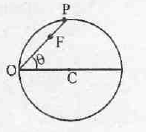Click to Chat

1800-1023-196

+91-120-4616500

CART 0

• 0

MY CART (5)

Use Coupon: CART20 and get 20% off on all online Study Material

ITEM
DETAILS
MRP
DISCOUNT
FINAL PRICE
Total Price: Rs.

There are no items in this cart.
Continue Shopping
```        A particle P is moving on a circle under the action of only one force acting always towards fixed point O on the circumference. Find ratio of////////////////ere dt2&de^2v dt j```
7 years ago

```							Dear Anurag,
Objects moving in circles have a speed which is equal to the distance traveled per time of travel. The distance around a circle is equivalent to a circumference and calculated as 2•pi•R where R is the radius. The time for one revolution around the circle is referred to as the period and denoted by the symbol T. Thus the average speed of an object in circular motion is given by the expression 2•pi•R / T. Often times the problem statement provides the rotational frequency in revolutions per minute or revolutions per second. Each revolution around the circle is equivalent to a circumference of distance. Thus, multiplying the rotational frequency by the circumference allows one to determine the average speed of the object.
The acceleration of objects moving in circles is based primarily upon a direction change. The actual acceleration rate is dependent upon how rapidly the direction is being change and is directly related to the speed and inversely related to the radius of the turn. It ends up that the acceleration is given by the expression v2 / R where v is the speed and R is the radius of the circle.
The equations for average speed (v) and average acceleration (a) are summarized below.
v = d / t = 2•pi•R / T = frequency • 2•pi•Ra = v2 / R
Directional Quantities for Objects Moving in Circles
A successful mathematical analysis of objects moving in circles is heavily dependent upon a conceptual understanding of the direction of the acceleration and net force vectors. Movement along a circular path requires a net force directed towards the center of the circle. At every point along the path, the net force must be directed inwards. While there may be an individual force pointing outward, there must be an inward force which overwhelms it in magnitude and meets the requirement for an inward net force. Since net force and acceleration are always in the same direction, the acceleration of objects moving in circles must also be directed inward.
Free Body Diagrams and Newton''s Second Law
Often times a force analysis must be conducted upon an object moving in circular motion. The goal of the analysis is either to determine the magnitude of an individual force acting upon the object or to use the values of individual forces to determine an acceleration. Like any force analysis problem, these problems should begin with the construction of a free-body diagram showing the type and direction of all forces acting upon the object. From the diagram, an Fnet = m•a equation can be written. When writing the equation, recall that the Fnet is the vector sum of all the individual forces. It is best written by adding all forces acting in the direction of the acceleration (inwards) and subtracting those which oppose it. Two examples are shown in the graphic below.Newton''s Law of Universal Gravitation
Orbiting satellites are simply projectiles - objects upon which the only force is gravity. The force which governs their motion is the force of gravitational attraction to the object which is at the center of their orbit. Planets orbit the sun as a result of the gravitational force of attraction to the sun. Natural moons orbit planets as a result of the gravitational force of attraction to the planet. Gravitation is a force which acts over large distances in such a manner that any two objects with mass will attract. Newton was the first to propose a theory to describe this universal mass attraction and to express it mathematically. The law, known as the law of universal gravitation states that the force of gravitational attraction is directly proportional to the product of the masses and inversely proportional to the square of the separation distance between their centers. In equation form,
Fgrav = G • m1 • m2 / d2
where m1 and m2 are the masses of the attracting objects (in kg) and d is the separation distance as measured from object center to object center (in meters) and G is the proportionality constant.
Cracking IIT just got more exciting,It s not just all about getting assistance from IITians, alongside Target Achievement and Rewards play an important role. ASKIITIANS has it all for you, wherein you get assistance only from IITians for your preparation and win by answering queries in the discussion forums. Reward points 5 + 15 for all those who upload their pic and download the ASKIITIANS Toolbar, just a simple  to download the toolbar….
So start the brain storming…. become a leader with Elite Expert League ASKIITIANS
Thanks
Aman Bansal

```
7 years ago
```								Since the Force is towards O. If one angle is ? other will also be ? because of isosceles triangle	F cos ? = mw2R	 F sin ?= m a  R	 tan ?=  a/ w2  = Y	 Y  is for 180-2? … we can differentiate to get it for ? ..	ANSWER is 2 tan ?.?
```
5 years ago
Think You Can Provide A Better Answer ?

## Other Related Questions on Mechanics

View all Questions »### Course Features

• 101 Video Lectures
• Revision Notes
• Previous Year Papers
• Mind Map
• Study Planner
• NCERT Solutions
• Discussion Forum
• Test paper with Video Solution### Course Features

• 110 Video Lectures
• Revision Notes
• Test paper with Video Solution
• Mind Map
• Study Planner
• NCERT Solutions
• Discussion Forum
• Previous Year Exam Questions